# Completing the square – getting rid of the square (difficult)

When it isn’t obvious how to get rid of the square

Complete the following square: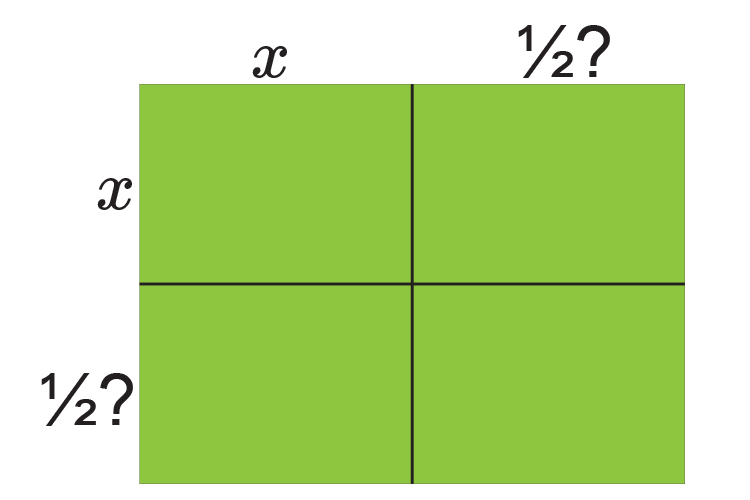Remember the above picture

NOTE:

1. The coefficient (or number) in front of the x  must be a 1
2. The next square must be ½ of the next term
3. Multiply out into all of the boxes

This method uses a visual model to solve a quadratic equation

For example:

x^2+2x-8=0

Visually x^2  can look like an area of a table: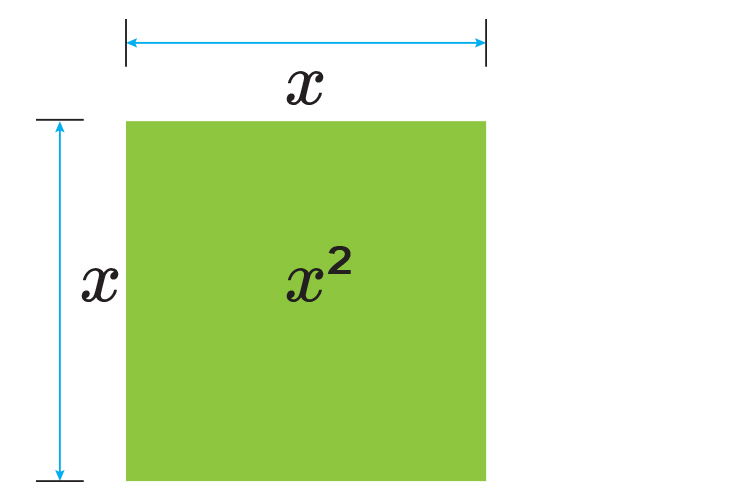The 2x  can be represented by: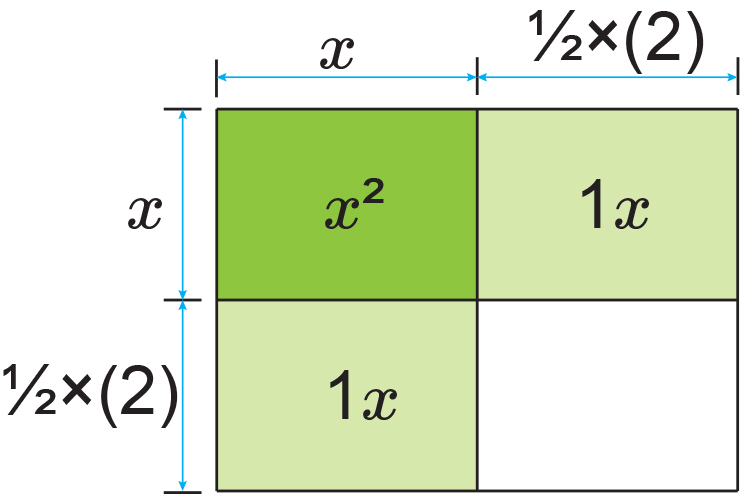And this will therefore complete the last square as: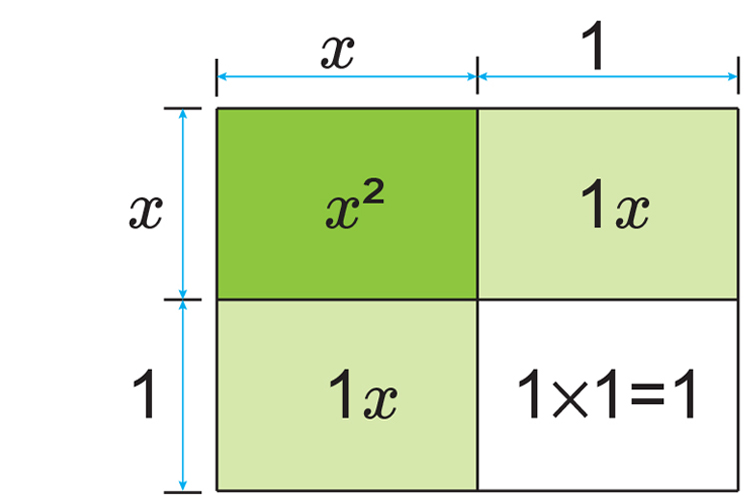NOTE:

This is the same as (x+1)^2

At the moment if you add each area together you get:

x^2+1x+1x+1times1

=x^2+2x+1

Originally we had  x^2+2x-8=0

We now have         x^2+2x+1=0

Always plot this on a number line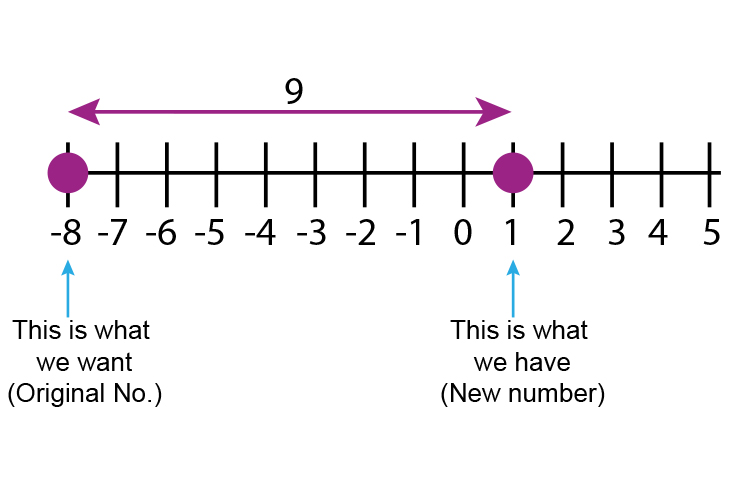Original number -  New number

-8-1=-9  (we need to -9 )

So x^2+2x-8=0

Is the same as (x+1)^2-9=0

Which can now be solved

(x+1)^2-9=0

(x+1)^2=9

x+1=+-sqrt9

(Don't forget the root of anything can be +  or -)

x+1=+-3

x=-1+-3

x=-1+3\ \ \ or\ \ \ x=-1-3

x=2\ \ \ or\ \ \ x=-4

## Now check

x^2+2x-8=0

If x=2            2^2+2times2-8=0  Which is correct

If x=-4     (-4)^2+2times(-4)-8=0

16-8-8=0  Which is correct

(If they don't add up to zero you can be assured that it is wrong)

The roots of x^2+2x-8=0  are x=2  and x=-4